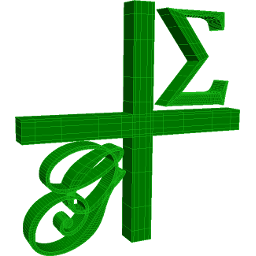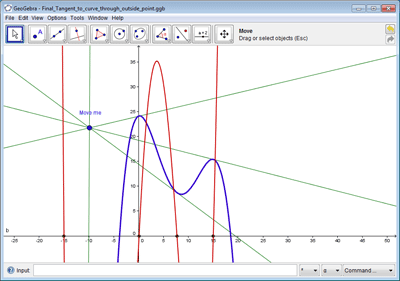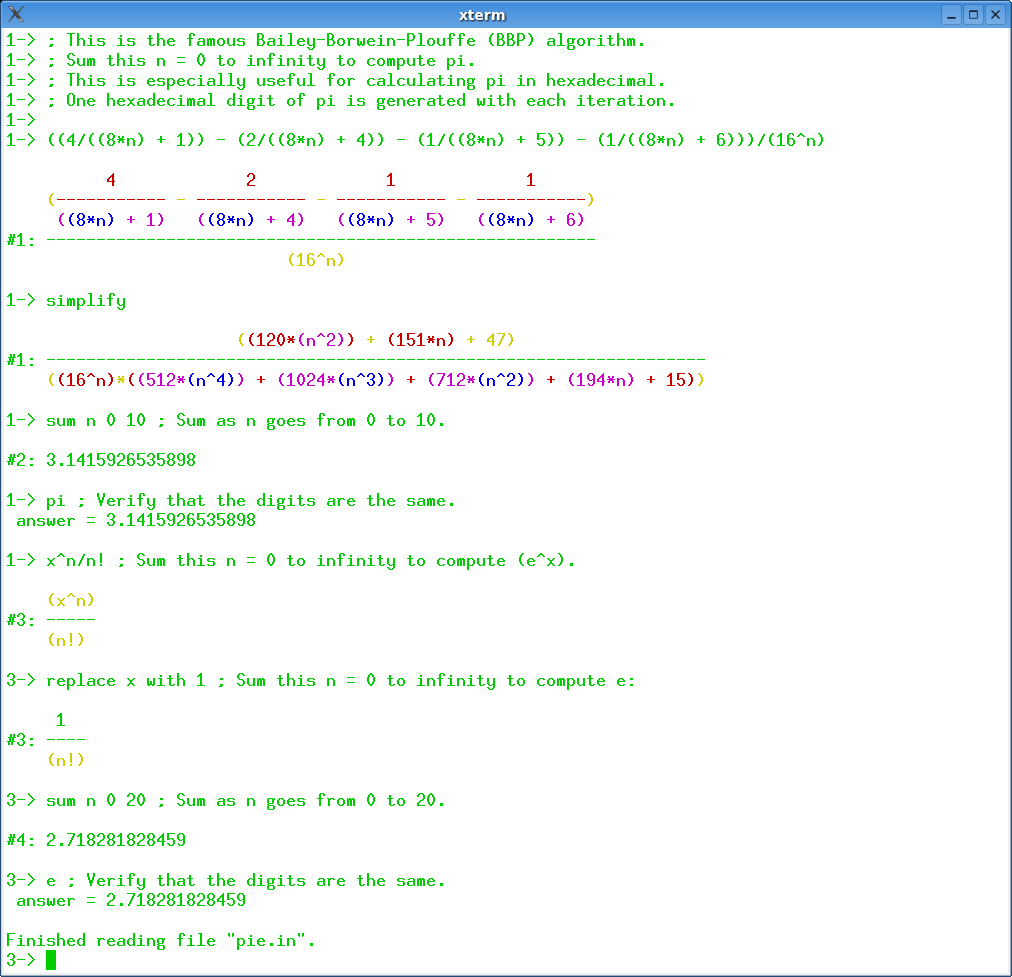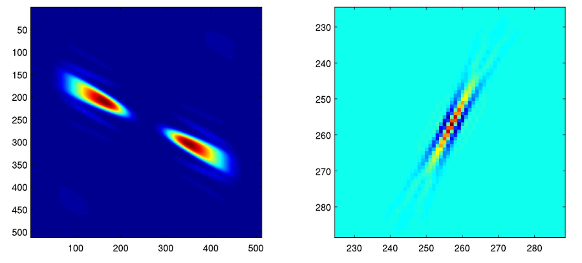18 Search Results

cdd/cddplus

The program cdd+ is a C++ implementation of the Double Description Method of Motzkin et al. for generating all vertices (i.e. extreme points) and extreme rays of a general convex polyhedron in R^d given by a system of linear inequalities.

Convex

Convex is a Maple package for convex geometry. It can deal with rational polytopes, cones and other polyhedra of arbitrary dimension. Polyhedral complexes and fans are also supported, as well as computations in face lattices. The integration into Maple makes Convex particularly suited for "applied" problems where polyhedra arise together with other mathematical structures.

emgr

Empirical gramians can be computed for linear and nonlinear control systems for purposes of model order reduction or system identification. Model reduction using empirical gramians can be applied to the state space, to the parameter space or to both through combined reduction. The emgr framework is a compact open source toolbox for (empirical) gramian-based model reduction and compatible with OCTAVE and MATLAB.

G+Smo

G+Smo (Geometry + Simulation Modules, pronounced "gismo") is a new open-source C++ library that brings together mathematical tools for geometric design and numerical simulation. It is developed mainly by researchers and PhD students. It implements the relatively new paradigm of isogeometric analysis, which suggests the use of a unified framework in the design and analysis pipeline. G+Smo is an object-oriented, cross-platform, template C++ library and follows the generic programming principle, with a focus on both efficiency and ease of use. The library is partitioned into smaller entities, called modules. Examples of available modules include the dimension-independent NURBS module, the data fitting and solid segmentation module, the PDE discretization module and the adaptive spline module, based on hierarchical splines of arbitrary dimension and polynomial degree. The library is licenced under the Mozilla Public License v2.0. It has been developed within the homonym research network supported by the Austrian Science Fund and aims at providing access to high quality, open-source software to the forming isogeometric numerical simulation community and beyond.

More informationGELDA

GELDA is a Fortran77 software package for the numerical integration of general linear differential-algebraic equations (DAE) with variable coefficients of arbitrary index. The implementation of GELDA is based on the construction of the discretization scheme, which first determines all the local invariants and then transforms the linear DAE into an equivalent strangeness-free DAE with the same solution set. The resulting strangeness-free system is allowed to have nonuniqueness in the solution set or inconsistency in the initial values or inhomogeneities. In the case that the DAE is found to be uniquely solvable, GELDA is able to compute a consistent initial value and apply the well-known integration schemes for DAEs. In GELDA the BDF methods and Runge-Kutta schemes are implemented.

GeoGebra

GeoGebra is free and multi-platform dynamic mathematics software for all levels of education that joins geometry, algebra, tables, graphing, statistics and calculus in one easy-to-use package. It has received several educational software awards in Europe and the USA.Quick Facts: * Graphics, algebra and tables are connected and fully dynamic * Easy-to-use interface, yet many powerful features * Authoring tool to create interactive learning materials as web pages * Available in many languages for our millions of users around the world * Free and open source software

More informationGEOMS

GEOMS is a Fortran77 software package for the numerical integration of general model equations (equations of motion) of multibody systems. The code combines a regularization technique with the Runge-Kutta method of type Radau IIa of order 5. This regularization technique reduces the index under preservation of all information of the solution manifold and, therefore, allows a robust numerical integration of the system.

GOBLIN Graph Library

A C++ class library including the whole bunch of standard algorithms in graph optimization and drawing. On top of this, a Tcl/Tk wrapper and a GUI for manipulating and editing of graphs.

HSL

HSL (formerly the Harwell Subroutine Library) is a collection of ISO Fortran codes for large scale scientific computation, written by members of the Numerical Analysis Group and other experts.

LattE integrale

LattE (Lattice point Enumeration) is a computer software dedicated to the problems of counting lattice points and integrationinside convex polytopes. LattE contains the first ever implementation of Barvinok's algorithm. The latest version, LattE integrale, has the ability to directly compute integrals of polynomial functions over polytopes and in particular to do exact volume computations. Version 1.6 added the capability of computing the highest coefficients of weighted Ehrhart quasipolynomials.

LiDIA

LiDIA is a C++ library for computational number theory which provides a collection of highly optimized implementations of various multiprecision data types and time-intensive algorithms.

LinBox

LinBox is a C++ template library for exact, high-performance linear algebra computation with dense, sparse, and structured matrices over the integers and over finite fields. LinBox has the following top-level functions: solve linear system, matrix rank, determinant, minimal polynomial, characteristic polynomial, Smith normal form and trace. A good collection of finite field and ring implementations is provided, for use with numerous black box matrix storage schemes.

Mathomatic

Mathomatic is a free, portable, general-purpose CAS (Computer Algebra System) and calculator software that can symbolically solve, simplify, combine, and compare equations, perform complex number and polynomial arithmetic, etc. It does some calculus and is very easy to use.

More informationrbMIT

The rbMIT © MIT software package implements in Matlab® all the general reduced basis algorithms. The rbMIT © MIT software package is intended to serve both (as Matlab® source) "Developers" — numerical analysts and computational tool-builders — who wish to further develop the methodology, and (as Matlab® "executables") "Users" — computational engineers and educators — who wish to rapidly apply the methodology to new applications. The rbMIT software package was awarded with the Springer Computational Science and Engineering Prize in 2009.

RCWA

RCWA is a package for the computer algebra system GAP. It provides implementations of algorithms and methods for computing in certain infinite permutation groups. The class of groups which RCWA in principle can deal with includes the finite groups, the free groups of finite rank, the free products of finitely many finite groups, certain infinite simple groups, certain divisible torsion groups and groups of many further types. It is closed under taking direct products and under taking wreath products with finite groups and with the infinite cyclic group (Z,+).

ShearLab 3D

ShearLab 3D is a MATLAB Library, developed for processing two- and three-dimensional signals using a certain class of basis functions named shearlets. These functions were developed with the goal of constructing systems of basis-functions nicely suited for representing anisotropic features (e.g. curvilinear singularities) that often are present in multivariate data. ShearLab 3D is optimized for GPU computing with CUDA and contains examples for applications like image and video denoising or image and video inpainting.

More informationSTATGRAPHICS Online

STATGRAPHICS Online is a statistical package that runs within a web browser. You can enter data directly into the data editor or read data from text files, Excel files, or other common formats. The calculations are performed remotely on a web server and the results returned to your browser as HTML with imbedded graphics images.